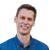# How do we find out the angle of a geometry (Rectangle) on a map.

600
3
08-23-2017 09:45 AMNew Contributor III

Trying to find out if it is possible to find out angle of a geometry: Rectangle geometry in my case.

Thanks,

Bikesh

Tags (2)
1 Solution

Accepted SolutionsbyEsri Frequent Contributor

A polygon doesn't really have an "angle". You could make the assumption that if your polygon has 4 vertices, it's a rectangle. Then you need to make an assumption about which vertex is at which index (ie if it's rotated 0 degrees, is index 0 the lower-left vertex?).

Once you do that, you could take two consecutive vertices and use trigonometry to calculate an angle.

So you would end up with something along the lines of this:

MapPoint firstVertex = polygon.Parts.StartPoint;
MapPoint secondVertex = polygon.Parts.EndPoint;
double xDiff = secondVertex.X - firstVertex.X;
double yDiff = secondVertex.Y - firstVertex.Y;
double angle =  Math.Atan2(yDiff, xDiff) * 180.0 / Math.PI;

3 RepliesbyEsri Frequent Contributor

A polygon doesn't really have an "angle". You could make the assumption that if your polygon has 4 vertices, it's a rectangle. Then you need to make an assumption about which vertex is at which index (ie if it's rotated 0 degrees, is index 0 the lower-left vertex?).

Once you do that, you could take two consecutive vertices and use trigonometry to calculate an angle.

So you would end up with something along the lines of this:

MapPoint firstVertex = polygon.Parts.StartPoint;
MapPoint secondVertex = polygon.Parts.EndPoint;
double xDiff = secondVertex.X - firstVertex.X;
double yDiff = secondVertex.Y - firstVertex.Y;
double angle =  Math.Atan2(yDiff, xDiff) * 180.0 / Math.PI;New Contributor III

Thank you Morten. That was really helpful. Do you know if there is a way to draw a rectangle given just length, width, centerpoint of rectangle and may be angle?

Thanks,

BikeshbyEsri Frequent Contributor

There's nothing out of the box, but you have everything needed to use a little trigonometry and math to figure out where the 4 vertices go.